### Knowing Linest Formula and 10 Different Examples in Excel

The Linest function is one of the benefits of using Microsoft Excel. The function is mainly for calculating statistics for a specific line, and it uses a method known as “least squares” when calculating the straight line that will perfectly fits the inputted data, and then it would return with the array that successfully describe that line. This is because Linest function will be returning with values of array, which makes it necessary for the data to be entered into the Linest formula.

Linest Formula Parameters

The Linest formula has four different components, which are known_y’s, followed by known_x’s, and then const, and finally the stats.

Known_y’s: This is a required part of the formula. A set of its values that are already known in a relationship, which means that the y = mx + b. If it happens that the component is one column, then every column in the one that is in known_x’s will be interpreted as a variable that is separated.

Known_x’s: This is an optional part of the formula. There is a set of x values that might already be known to be in a relationship, which means y = mx + b. This is a range that has the opportunity of including either one or more sets of different variables. If it is only a single variable that is being used, then both the known_y’s & _x’s have the possibilities of being in any shape. But it is only if they both have an equal range. If it happens that more than one variable is being used, then a known_y’s must be seen as a vector, but it is only if a range is either a row or width of column. If this known_x’s has been omitted, then it will automatically be assumed to be array that has the very same size as the previous component (known_x’s).

Const: This is optional. It is the logical value that will specify where or not to force constant b to be equal to 0. If this has been set to true or omitted, then b will normally be calculated. If it is set to false, then b would be set equal to 0, which makes the m-values to be adjusted, so it would fit a condition, where y = mx.

Stats: This is final and optional. It is the logical value that will specify whether it will return the statistic that has additional regression. If this has been set to true, linest will be returning that statistic. If it has been set to false or even omitted, it would then only return with m-coefficients and a constant b.

Example 1: Simple Linest Formula

We needs to find a statistic data for the number of people whom were available with linest formula. This example is about the simplest linest formula.I need a statistic data about the number of people as they have been divided in ages. This example provides the possibility of finding an improved answer, and we are going to use a complete linest formula to get the statistic information that we strives after, and we are going to use the same data that we have used in previous example.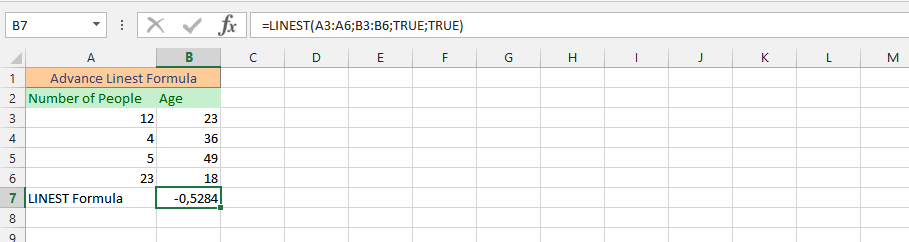Example 3: SUM and LINEST Formulas Simultaneously

The business is going to a specific level, because we have made certain sales that we have already set for ourselves for the year. But, we want to make some adjustments to make an estimation of how many sales we can make in the upcoming month. We have gotten more ambitious in our business, which is why we need to find out if a made estimation is achievable. Our estimation is 15000. This is where the sum and linest formula is being used to help us to determine if that goal is achievable.Example 4: Linest Formula with Text

In this example, we have layout our data, and it is very important that we use text rather than cells, because we have already given them a respective label.Example 5: Average Performance with Linest Formula

This example is perfectly suitable for informing that under the circumstances that we have already set an average goal that every six week should yield, we need to also use the average formula in combination to the linest formula, so we can get a statistic value of the average performance.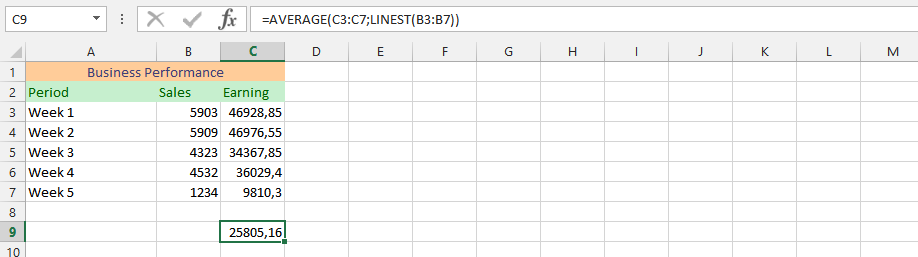Example 6: Using both XOR and Linest Formula

We have set a specific goal for the business, and we want to know if this would be true or false. This is why we use both the xor and linest formula simultaneously to find out if it would be true or false.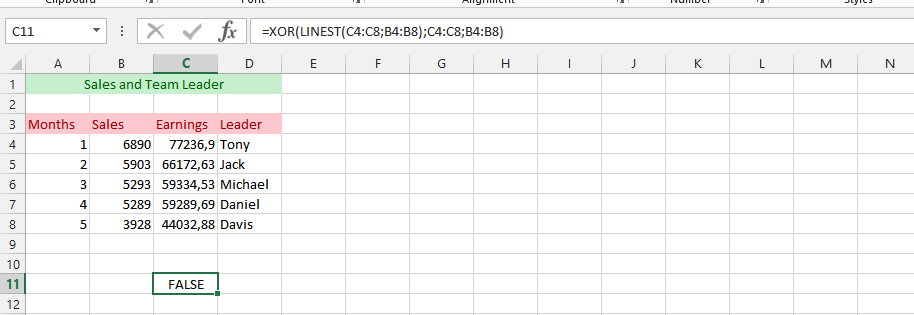Example 7: MMULT and LINEST Formula Simultaneous

Usage In this example, we need a matrix Value and statistic value for the outlaid data. We are going to use both the MMULT formula with linest formula to find the simultaneous value.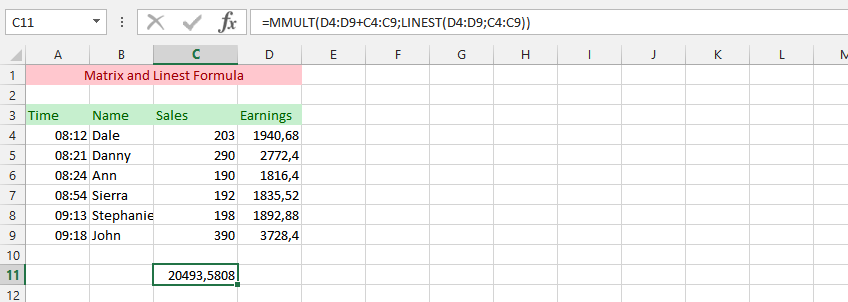Example 8: SUMPRODUCT & LINEST Formula Simultaneously

In this example, we are using the same data as the previous one, but this time we are using both the sumproduct and linest formula to find the answer that have eluded us for the period of time. This is something that will make it possible for us to make the right assessments for the data that has been inputted, and see the differences in the formula.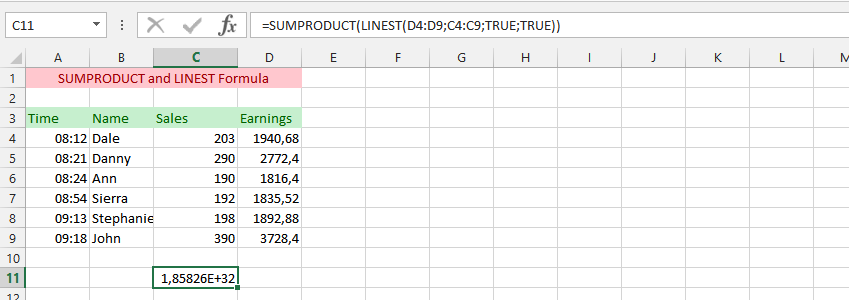Example 9: Linest on Different Spreadsheet

The example is based on finding a statistic value with the linest formula that has been placed on a different spreadsheet that makes it possible to be more flexible in the details that have been provided, under the circumstances that we might need to make some adjustment in the future.Example 10: What about another Document?

We are going to use the same data as previous one, but this time we are going to use them on another document. This is about assessing that it will gives the same result that is showing in the previous example, and provides you the opportunity of verifying that it is very possible to use the linest formula using data from a different data.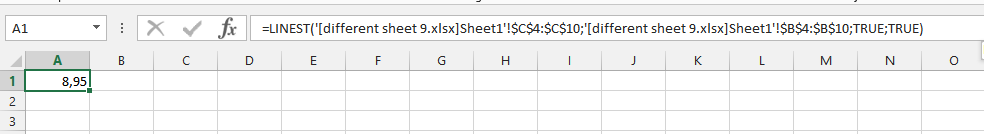Previous articleAvoid Errors Using IFERROR-Everyone Should Know
Next articleLinking Text Box To A Specific Cell